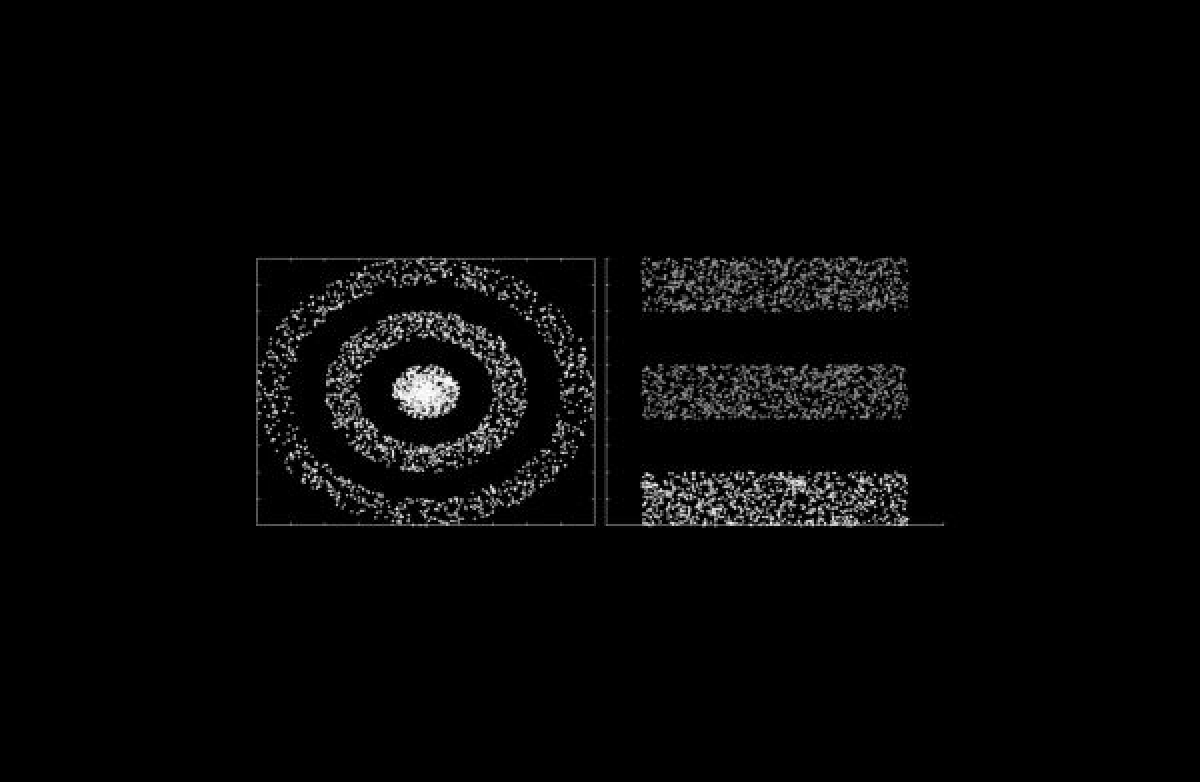# Improving Quantum K-Means

Arvind S. Menon, Nikaash Puri

,

December 13, 2022Arvind S. Menon & Nikaash Puri (Adobe India)

Many quantum machine learning algorithms draw inspiration out of their classical analogues and attempt at solving the same problem with the help of quantum computing. Q-means is one such algorithm which is the quantum analogue of the classical K-means algorithm. K-Means is an unsupervised learning algorithm in machine learning, which groups the unlabeled dataset into different clusters, thus discovering the categories of groups in the unlabeled dataset. On the other hand, Q-means is implemented on the quantum states to obtain characteristic cluster quantum states, i.e. the quantum states that are in a superposition of all the quantum states in a particular cluster. This is done by evaluating a cost function based on the cluster quantum states through measuring the overlap or fidelity between the characteristic cluster quantum states, ensuring high separation between clusters and low cluster scatter about the centroids.

Early implementations of Q-means claim an exponential speedup over its classical counterpart, which sounds very promising since this is a NP-Hard problem for d-dimensional observations; however, this speedup is only possible for linearly separable clusters. Q-means performs cluster assignment based on Euclidean distances, which does not work for non-linearly separable clusters in the original space, which can be a significant limitation. Therefore, there is a need for feature mapping / kernel methods to efficiently separate the data in a high dimensional space, which is closely related to the Support Vector Machine (SVM) method of operation.

This paper proposes a hybrid quantum-classical algorithm that learns a suitable quantum feature map which separates non linearly separable unlabeled data using a variational quantum feature map and Q-means as a subroutine for unsupervised learning. The objective of the paper is the proposal of the new hybrid algorithm that includes Q-means. The objective of the algorithm is to maximally separate the clusters in the quantum feature Hilbert space.

The quantum circuit involves encoding unlabeled classical data using quantum gates that perform unitary operations on the initial quantum state based on quantum parameters and input data. To perform quantum feature encoding, 1) a variational circuit is used to learn the best possible quantum feature map that obtains meaningful clustering results. Since the quantum feature Hilbert space dimensions are exponential with respect to qubits used in the initial state, high dimensional feature spaces can be efficiently implemented. 2) Kernel estimation and cluster assignment is then performed by implementing the Q-means sub-routine. 3) Finally, measurement is done to compute the overlap between the characteristic cluster quantum states, that also acts as a measure of separation between the clusters. 4) The output of the complete quantum circuit is used to compute the value of the cost function that is based on the Hilbert-Schmidt distance between the density matrices of the characteristic cluster quantum states.

The simulations were implemented to compute overlap cost function using Pennylane’s QAOA embedding framework. Sk-learn is used to pre-process all the datasets before quantum embedding. Cluster datasets with labels were used as inputs for the quantum embedding circuit to train the quantum parameters. For the considered 3 datasets; blobs, concentric circles and moons, the algorithm took the least iterations to converge for the blobs as they are very well separated in the original space, whereas in the case of non-linearly separable datasets, the algorithm took a larger number of iterations to converge.

The overall method adapts a repetitive approach of performing unsupervised learning followed by adaptively training the feature map, which aims at learning the best representation of the data in the quantum feature Hilbert space. It is shown that one can explore more feature spaces that are better suited for the embedded dataset by explicitly implementing the kernel estimation. However, since there are no quantum simulators available to test Q-means to date, the work could not establish quantum advantage experimentally.